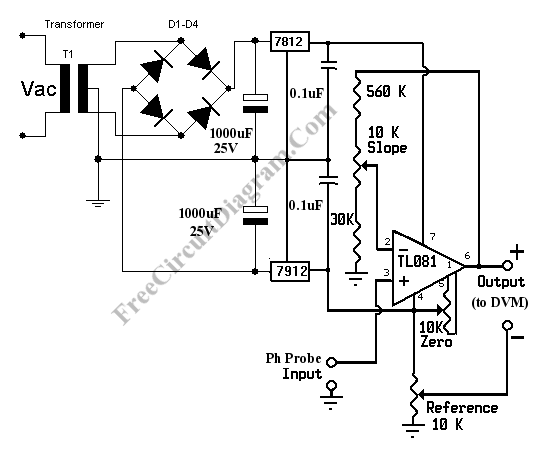# Simple pH MeterpH Meter Circuit

pH meter measures the voltage (the EMF, electromotive force) generated by the pH electrodes (the sensor) to show the hydrogen concentration in pH scale. Basically, a pH meter is nothing more than a pH probe/sensor with high impedance voltmeter that measures the generated voltage at the pH probe. To measure the pH with a standard digital voltmeter (DVM), all you need is just a pre-amplifier that provide the high impedance interface with the gain that match the pH-Voltage conversion factor. Here is one example of pH meter circuit diagram:pH Meter Calibration

Before the pH meter can be used, it should be calibrated first. Here is the steps to calibrate this pH meter circuit:

• Null the amplifier by shorting the pH probe input to ground. Measure the voltage between output + and ground. Adjust the zero potentiometer until the voltmeter reading show zero volt (or close to zero).
• Now connect pH probe  input to a 413 mV voltage source. You can use a potentiometer to make a  simple 413 mV voltage source. After connecting the input to 413 mV source, then measure the  + output  and ground using the digital voltmeter  and you should read 7.00 volt. If not then adjust the slope potentiometer until you get the closest reading to 7.00 volts.
• Short the pH probe input to ground. Connect the digital voltmeter voltmeter between – output and + output. Now adjust reference potentiometer until the voltmeter show 7.00 volts. This corresponds to the neutral pH of 7.
• Connect the pH probe input to a pH electrodes (pH probe/sensor), now the pH meter is ready to use. The voltmeter reading in volts would be direct conversion of pH. pH value of 7 would be read as 7 volts, pH 3 as 3 volts, etc.

[Source: simplecircuitdiagram.com]